# Average Quiz Quantitative Aptitude Quiz for IBPS , SBI , RBI Prelims | Quiz – 4

## Age Based Quiz Quantitative Aptitude Quiz for IBPS , SBI , RBI Prelims : Quiz – 4

Questions based on average can be found in a variety of competitive exams. The method required to solve the questions on Average must be acquainted to the candidates. We’ve created the Average Based Quiz in this article to help you prepare for forthcoming exams. This Average Based Quiz comprises all of the most recent pattern-based questions from the IBPS, SBI, and RBI PO and Clerk exams, as well as previous year average questions. This Average Based Quiz is completely free. Each question in this Average Based Quiz has a full explanation. This Average Based Quiz can help you study for forthcoming examinations such as banking, insurance, SSC, and railway.

1. The average marks in Maths of a class of 25 students is 70. If the marks of two students were misread as 42 and 64 instead of the actual marks 68 and 58 respectively, then what would be the correct average?
(a) 70.8
(b) 69.6
(c) 69.8
(d) 70.4
(e) 70.2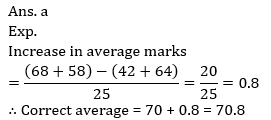2. If the average marks of 30 boys in a class is 45 and the total marks scored by all student together is 2800. Then find the average marks scored by girls if total student in class is 50?
(a) 70
(b) 72.5
(c) 75
(d) 82
(e) 84.53. In three numbers, the first is twice the second and thrice the third. If the average of three numbers is 99, then the first number is
(a) 165
(b) 162
(c) 164
(d) 169
(e) None of these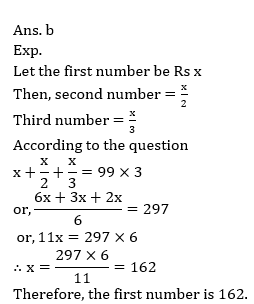4. The average of five positive numbers is 470. The average of first two numbers is 720 and the average of the last two numbers is 150. What is the third number?
(a) 610
(b) 600
(c) 510
(d) 500
(e) None of these5. A, B, C, D and E are five consecutives even numbers. Average of A and E is 46. What is the largest number?
(a) 52
(b) 42
(c) 50
(d) 48
(e) None of these6. The average of seven numbers is 21. If the average of first three numbers is 19 and that of last three numbers is 20, what is the fourth number from starting?
(a) 35
(b) 32
(c) 30
(d) 40
(e) None of these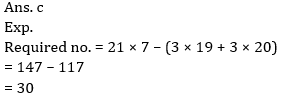7. After replacing an old member by a new member, it was found that the average age of five members of a club is the same as it was 3 years ago. The difference between the ages of the replaced and the new member is :
(a) 2 years
(b) 4 years
(c) 8 years
(d) 15 years
(e) None of these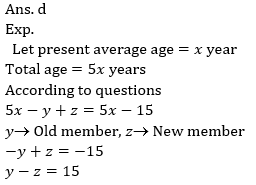8.The average temperature from Monday to Wednesday is 37°C while the average temperature from Tuesday to Thursday is 34°C. The temperature of Thursday is 4/5 times to that of Monday. Find the temperature of thursday?
(a)36°C
(b)33°C
(c)37°C
(d)34°C
(e)None of these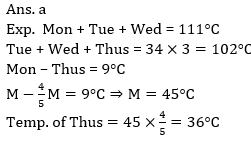9. The average score of 55 students of a class is 88. If top five students are removed, the average drops by 5 marks. If the five toppers’ marks are consecutive decreasing integers, then find the toppers score.
(a) 140
(b) 150
(c) 145
(d) 155
(e) None of these10. In an examination, the average marks obtained by the students was found to be 60. After omission of computational errors, the average marks of 100 candidates had to be changed from 60 to 30 and the average with respect to all the examinees came down to 45 marks. The total number of candidates who took the exam, was:
(a) 200
(b) 210
(c) 240
(d) 180
(e) None of these#### Attempt Quantitative Aptitude Topic Wise Online Test Series

Recommended PDF’s for :

#### Most important PDF’s for Bank, SSC, Railway and Other Government Exam : Download PDF Now

AATMA-NIRBHAR Series- Static GK/Awareness Practice Ebook PDF Get PDF here
The Banking Awareness 500 MCQs E-book| Bilingual (Hindi + English) Get PDF here
AATMA-NIRBHAR Series- Banking Awareness Practice Ebook PDF Get PDF here
Computer Awareness Capsule 2.O Get PDF here
AATMA-NIRBHAR Series Quantitative Aptitude Topic-Wise PDF Get PDF here
AATMA-NIRBHAR Series Reasoning Topic-Wise PDF Get PDF Here
Memory Based Puzzle E-book | 2016-19 Exams Covered Get PDF here
Caselet Data Interpretation 200 Questions Get PDF here
Puzzle & Seating Arrangement E-Book for BANK PO MAINS (Vol-1) Get PDF here
ARITHMETIC DATA INTERPRETATION 2.O E-book Get PDF here
3